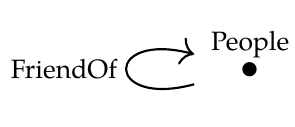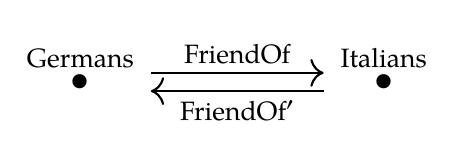To prepare yourself for the next phase of the course, here are some puzzles illustrating the ideas in [Lecture 41](https://forum.azimuthproject.org/discussion/2230/lecture-41-chapter-3-composing-functors/p1).

Let's transform a database by composing it with a functor! We already did this in [Lecture 41](https://forum.azimuthproject.org/discussion/2230/lecture-41-chapter-3-composing-functors/p1), and the answer should have made a lot of sense. But this time the answer will be strange... leading us to the next phase of the course.

**Puzzle 122.** Let \$$\mathcal{C}\$$ be the free category on this graph:Let's use this as a database schema. Draw an example of a database built using this schema. Mathematically it's a functor \$$F: \mathcal{C} \to \mathbf{Set}\$$. But what does this mean in concrete terms? You can draw it as a table, like you would see in a spreadsheet.

**Puzzle 123.** Let \$$\mathcal{D}\$$ be the free category on this graph:Again this can be used as a database schema. What does a database built using this schema amount to, in concrete terms?

**Puzzle 124.** Show there exists a unique functor \$$G : \mathcal{D} \to \mathcal{C}\$$ with

$G(\textrm{FriendsOf}) = G(\textrm{FriendsOf}^\prime) = \textrm{FriendsOf} .$

What does this functor do, in concrete terms?

**Puzzle 125.** Take the database \$$F: \mathcal{C} \to \mathbf{Set}\$$ that you constructed in Puzzle 122 and form the database \$$F \circ G : \mathcal{D} \to \mathbf{Set}\$$. What do you get?

Don't be shocked if the answer to this last puzzle is sort of weird. You are taking a database about \$$\mathrm{People}\$$ and their friends and turning it into a database about \$$\mathrm{Germans}\$$ and \$$\mathrm{Italians}\$$... without having any information at hand about which people are Germans and which are Italians! This is bound to give something strange.

A more reasonable thing would be this: we start out with a database about \$$\mathrm{Germans}\$$ and \$$\mathrm{Italians}\$$, forget who is German and who is Italian, and get a database about \$$\mathrm{People}\$$.

This means starting with a functor from \$$\mathcal{D}\$$ to \$$\mathbf{Set}\$$ and getting a functor from \$$\mathcal{C}\$$ to \$$\mathbf{Set}\$$. And this is a bit more tricky than what we just did, namely going the other way. We need to do something called a ['Kan extension'](https://en.wikipedia.org/wiki/Kan_extension).

Kan extensions are great. In his book _Categories for the Working Mathematician_, Saunders Mac Lane wrote:

> The notion of Kan extensions subsumes all the other fundamental concepts of category theory.

So, I'll have to explain Kan extensions!

But for this I'll need to explain two other concepts: ['natural transformations'](https://en.wikipedia.org/wiki/Natural_transformation) and ['adjoint functors'](https://en.wikipedia.org/wiki/Adjoint_functors). These are two of the most important concepts in category theory... and they're great fun.

**[To read other lectures go here.](http://www.azimuthproject.org/azimuth/show/Applied+Category+Theory#Chapter_3)**